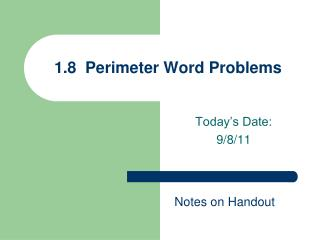Download Presentation1.8 Perimeter Word Problems

# 1.8 Perimeter Word Problems

Télécharger la présentation## 1.8 Perimeter Word Problems

- - - - - - - - - - - - - - - - - - - - - - - - - - - E N D - - - - - - - - - - - - - - - - - - - - - - - - - - -
##### Presentation Transcript

1. 1.8 Perimeter Word Problems Today’s Date: 9/8/11 Notes on Handout

2. Perimeter Rectangle: l w w Perimeter = l + l + w + w = 2l + 2w l Triangle: a b Perimeter = a + b + c c

3. Word Problems The perimeter of a triangle “is” 72. “is” means = a + b + c = 72 A length is 3 less “than” the width. “than” means switch the order l = w – 3 with subtraction, order matters l = 3 – w WRONG!!

4. Word Problems On the TEST: a) Define the variable b) Write the equation c) Solve W = width L = length 2(w + 5) + 2w = 170 width = 40 feet length = 45 feet

5. Examplesa) Define variable b) Write eqtn. c) Solve 1) The length of a rectangle is 5 inches more than the width. The perimeter is 34 inches. Find the length and width. l = w + 5 l= (w + 5) a) l = length w = width = 34 2l + 2w 2l+ 2w b) 2(w + 5) + 2w = 34 2w + 10 + 2w = 34 4w + 10 = 34 4w = 24 w = 6 • width = 6 in. • length = 11 in. = 11 l = 6 + 5

6. Examples a) Define variable b) Write eqtn. c) Solve 2) The length of a rectangle is 5 inches more than twice the width. The perimeter is 34 inches. Find the length and width. l= (2w + 5) l = 2w + 5 a) l = length w = width = 34 2l + 2w 2l+ 2w b) 2(2w + 5) + 2w = 34 4w + 10 + 2w = 34 6w + 10 = 34 6w = 24 w = 4 • width = 4 in. • length = 13 in. l = 2(4) + 5 = 8 + 5 = 13

7. Examplesa) Define variable b) Write eqtn. c) Solve 2nd side 3) One side of a triangle is twice the shortest side. The third side is 3 feet more than the shortest side. The perimeter is 19 feet. Find all three sides. b = 2a b = 2a • a = shortest side • b = 2nd side • c = 3rd side c = a + 3 c = a + 3 a + b + c = 19 a + b + c = 19 c) 1st side = 4 ft. 2nd side = 8 ft. 3rd side = 7 ft. b) a + 2a + a + 3 = 19 4a + 3 = 19 4a = 16 a = 4 b a b = 2(4) = 8 c c = 4 + 3 = 7

8. Homework #6 “Ant” Worksheet **Do on a Separate Paper** Show all work for a stamp!